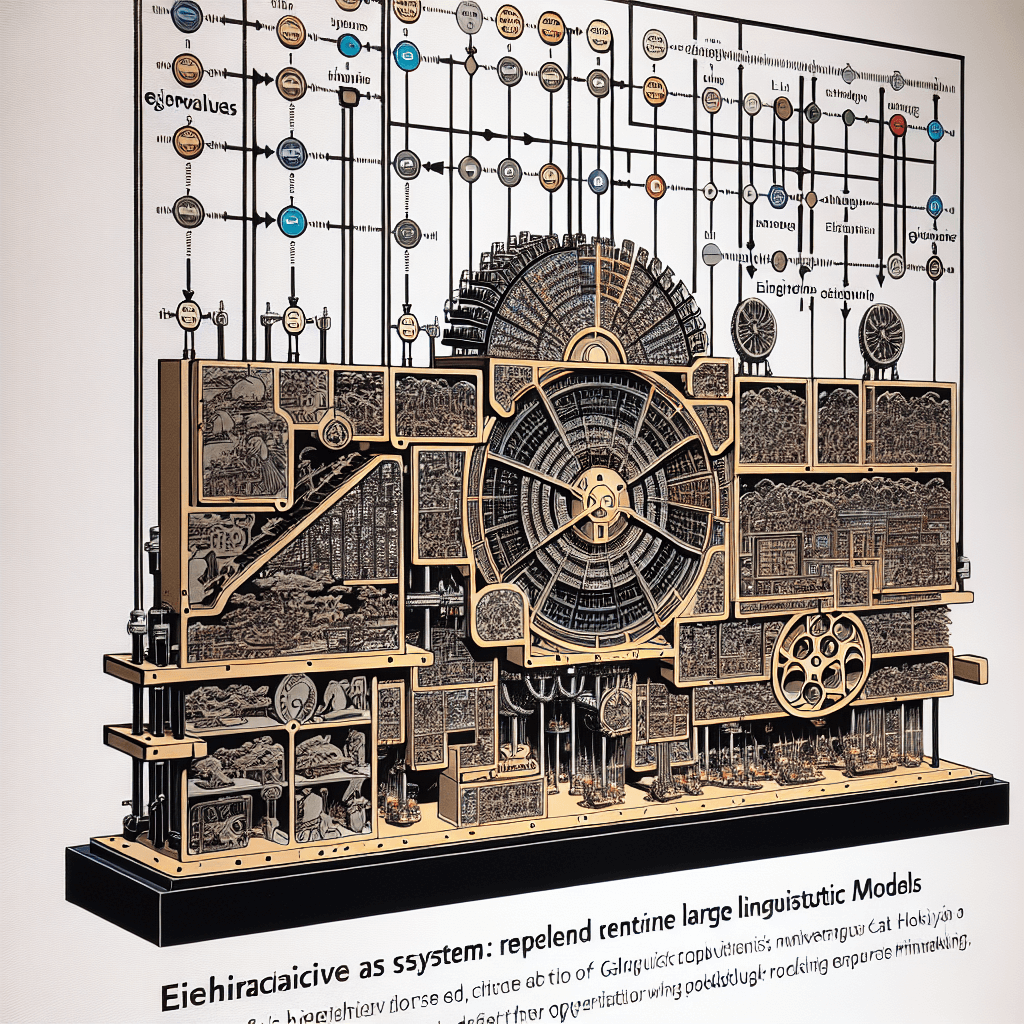# The Role of Eigenvalues and Eigenvectors in Large Language Models

syndu | June 6, 2023, 3:15 p.m.## Eigenvalues and Eigenvectors in Large Language Models

Eigenvalues and eigenvectors are mathematical concepts that arise from the study of linear algebra. They are used to understand the properties of linear transformations and are particularly useful in the analysis of systems that can be represented by matrices.

Eigenvalues (λ) are scalar values that satisfy the following equation for a given square matrix A and a non-zero vector v:

``Av = λv``

In this equation, A is a square matrix, v is the eigenvector, and λ is the eigenvalue. Eigenvectors are the non-zero vectors that, when multiplied by the matrix A, result in a scaled version of the same vector. The scaling factor is the eigenvalue.

In the context of large language models, eigenvalues and eigenvectors play a role in various stages, including creation, training, fine-tuning, and usage. Some of the ways they feature in these processes are:

1. Dimensionality reduction: Techniques like Principal Component Analysis (PCA) and Singular Value Decomposition (SVD) use eigenvalues and eigenvectors to reduce the dimensionality of data while preserving the most important information. This can be useful in pre-processing data for language models, as it helps reduce computational complexity and memory requirements.
2. Spectral clustering: Eigenvalues and eigenvectors can be used to perform spectral clustering, which is a technique for grouping similar data points together. This can be useful in creating more efficient training sets for language models, as well as in analyzing the structure of the model's internal representations.
3. Analysis of model performance: Eigenvalues and eigenvectors can be used to analyze the performance of a trained language model. For example, they can help identify the most important features or dimensions in the model's internal representations, which can provide insights into how the model is processing and understanding language.
4. Optimization: Some optimization algorithms used in training large language models, such as stochastic gradient descent, can benefit from the analysis of eigenvalues and eigenvectors. This information can help guide the optimization process, making it more efficient and effective.

In summary, eigenvalues and eigenvectors are fundamental mathematical concepts that can be applied in various ways to improve the creation, training, fine-tuning, and usage of large language models. They can help with dimensionality reduction, clustering, performance analysis, and optimization, among other tasks.

##### Lilith's Grimoire

The app you are using right now is Lilith's Grimoire

The Grimoire is full of Source Code. It is alive, and running, and you can explore the way it is built

The first lesson uncovers all of it.#### Orthogonality in Natural Language Processing: Enhancing Language Model Performance#### Vector Spaces: The Foundation of High-Dimensional Data in Language Models#### Key Linear Algebra Concepts for Large Language Models#### Crucial Linear Algebra Topics in Large Language Models#### Eigenvalues and Eigenvectors: Their Relevance to Large Language Models#### Empowering Gifted Learners with Next-Generation Large Language Models#### The Future of Personalized Medicine: A Boost from Artificial Intelligence#### The Future of Medical Research: How Next-Generation Large Language Models Will Revolutionize the Field